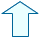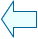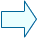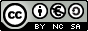Mass Metres, litres, kilograms

1 a . The kilogram.
The base unit of mass is the kilogram.
The base unit of mass is defined as the mass of one litre of water contained in a cubic decimetre.
The kilogram is the mass of  one litre of pure water at 4ºC. A cylinder with such a mass was made from an alloy of platinum and iridium. Since then, this cylinder has been the universal mass standard.
Esta unidad interactiva requiere la máquina virtual de Java J2RE.

 You can see the level of the containing water by dragging the pointer up on the container.

1 b . Multiples and submultiples of the kilogram.

The base unit of mass is the kilogram; it isn't the gram, for that reason the hectogram, decagram and the gram are submultiples of the kilogram.

The multiple of the kilogram is the metric tonne, which is 1000 kilograms.
A cubic metre of pure water weighs a tonne.

 Name of the unit Symbol kilogram base unit kg submultiples hectogram equals 0.1 kilograms hg decagram equals 0.01 kilograms dag gram equals 0.001 kilograms g decigram equals 0.0001 kilograms dg centigram equals 0.00001 kilograms cg milligram equals 0.000001 kilograms mg
Esta unidad interactiva requiere la máquina virtual de Java J2RE.
 Here, the units are out of order, you have to put them in descending order by dragging them to the top.Eduardo Barbero CorralSpanish Ministry of Education. Year 2007